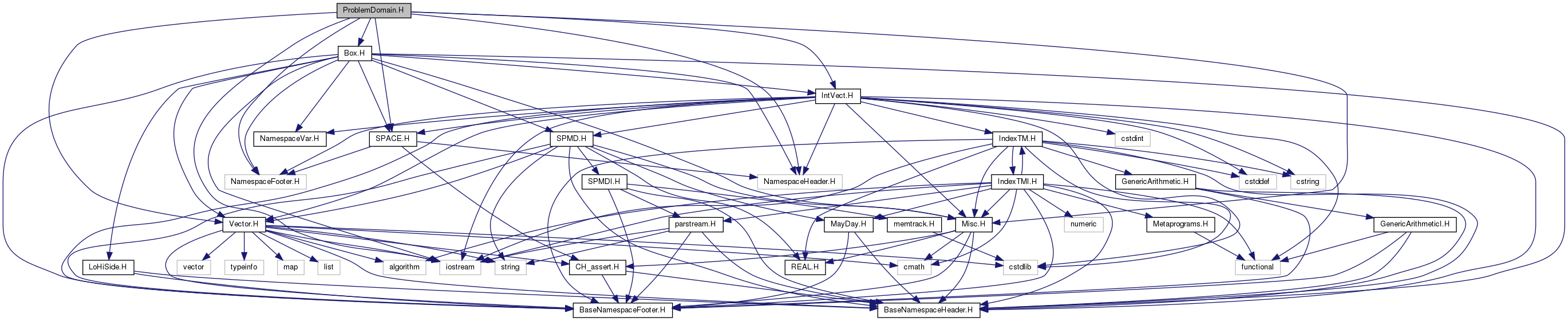Chombo + EB  3.2
ProblemDomain.H File Reference
`#include <iostream>`
`#include "Vector.H"`
`#include "IntVect.H"`
`#include "Box.H"`
`#include "Misc.H"`
`#include "SPACE.H"`
`#include "NamespaceHeader.H"`
`#include "NamespaceFooter.H"`
Include dependency graph for ProblemDomain.H:Go to the source code of this file.

## Classes

class  ShiftIterator
Class to manage box-shifting used to enforce periodic BC's. More...

class  ProblemDomain
A class to facilitate interaction with physical boundary conditions. More...

class  ImageIterator

## Macros

#define _PROBLEMDOMAIN_H_

## Functions

ProblemDomain grow (const ProblemDomain &pd, int i)

ProblemDomain grow (const ProblemDomain &pd, const IntVect &v)

Box bdryLo (const ProblemDomain &a_pd, int a_dir, int a_len=1)
Returns a face-centered Box at the low side of a_pd. More...

Box bdryHi (const ProblemDomain &a_pd, int a_dir, int a_len=1)
Returns a face-centered Box at the high side of a_pd. More...

Box adjCellLo (const ProblemDomain &a_pd, int a_dir, int a_len=1)
Returns the cell-centered Box adjacent to the low side of a_pd. More...

Box adjCellHi (const ProblemDomain &a_pd, int a_dir, int a_len=1)
Returns the cell-centered Box adjacent to the low side of a_pd. More...

## ◆ _PROBLEMDOMAIN_H_

 #define _PROBLEMDOMAIN_H_

## ◆ grow() [1/2]

 ProblemDomain grow ( const ProblemDomain & pd, int i )
inline

## ◆ grow() [2/2]

 ProblemDomain grow ( const ProblemDomain & pd, const IntVect & v )
inline

Returns a ProblemDomain that is the argument ProblemDomain with a DomainBox grown by the given amount.

References ProblemDomain::grow().

## ◆ bdryLo()

 Box bdryLo ( const ProblemDomain & a_pd, int a_dir, int a_len = `1` )

Returns a face-centered Box at the low side of a_pd.

Returns the edge-centered Box (in direction a_dir) defining the low side of the argument ProblemDomain. The output Box will have the given length in the given direction. Directions are zero-based. It is an error if not 0 <= dir < SpaceDim. The neighbor of an Empty ProblemDomain is an Empty Box of the appropriate type. If a_dir is a periodic direction, will return an empty Box.

Referenced by ProblemDomain::contains_box().

## ◆ bdryHi()

 Box bdryHi ( const ProblemDomain & a_pd, int a_dir, int a_len = `1` )

Returns a face-centered Box at the high side of a_pd.

Returns the edge-centered Box (in direction a_dir) defining the high side of the argument ProblemDomain. The return Box will have the given length in the given direction. Directions are zero-based. It is an error if not 0 <= dir < SpaceDim. The neighbor of an Empty ProblemDomain is an Empty Box of the appropriate type. If a_dir is a periodic direction, will return an empty Box.

Referenced by ProblemDomain::contains_box().

 Box adjCellLo ( const ProblemDomain & a_pd, int a_dir, int a_len = `1` )

Returns the cell-centered Box adjacent to the low side of a_pd.

Returns the cell centered Box of the given length adjacent to the argument ProblemDomain on the low end along the given coordinate direction. The return Box is identical to the argument ProblemDomain in the other directions. The return ProblemDomain and the argument ProblemDomain have an empty intersection.

NOTES:

• len >= 1.
• Box retval = adjCellLo(b,dir,len) is equivalent to the following set of operations:
Box retval(b);
retval.convert(dir,ProblemDomain::CELL);
retval.setrange(dir,retval.smallEnd(dir)-len,len);

Directions are zero-based. It is an error if not 0 <= dir < SpaceDim. The neighbor of an Empty ProblemDomain is an Empty Box of the appropriate type. If a_dir is a periodic direction, will return an empty Box as well.

Referenced by ProblemDomain::contains_box().

 Box adjCellHi ( const ProblemDomain & a_pd, int a_dir, int a_len = `1` )

Returns the cell-centered Box adjacent to the low side of a_pd.

Returns the cell centered Box of the given length adjacent to the argument ProblemDomain on the high end along the given coordinate direction. The return Box is identical to the argument ProblemDomain in the other directions. The return Box and the argument ProblemDomain have an empty intersection.

NOTES:

• len >= 1.
• Box retval = adjCellHi(b,dir,len) is equivalent to the following set of operations:
Box retval(b);
retval.convert(dir,ProblemDomain::CELL);
retval.setrange(dir,retval.bigEnd(dir)+1,len);

Directions are zero-based. It is an error if not 0 <= dir < SpaceDim. The neighbor of an Empty ProblemDomain is an Empty Box of the appropriate type. If a_dir is a periodic direction, will return an empty Box.

Referenced by ProblemDomain::contains_box().